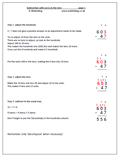# Resource of the Week: written subtractionThis week I am looking at one of the hardest ideas to get across to children; carrying out a subtraction which requires adjusting across two columns. This only happens when there is a zero involved.

For example:

703

286 –

3 – 6 does not give a positive answer so an adjustment to the top number needs to be made. A ten can not be borrowed as there are none.

The procedure then is to borrow from the hundreds, move to the tens and then move again to the units. Often children will borrow from the hundreds, ignore the zero in the tens and move straight to the units. They cross out the 7 and make it 6 and make the 3 into 13.

This does not work as it has been 100 borrowed, not 10. Extra steps need to be taken by making the tens column 10 and then taking one ten, making this 9, and adjusting to the units.

Some children will see a quicker way: making the 70 one less (69) and adjusting to the units.

More explanation is available on the worksheet.

Standard subtraction with zeros in the tens (pg 1)